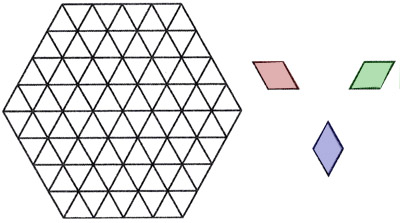BrainDen.com - Brain Teasers

## Question

A regular hexagon is divided into 2n equilateral triangles.
Pairing triangles that share an edge produces diamond shapes with three distinct orientations, as shown.Prove that any n-diamond tiling of the hexagon will use the three types in equal numbers.

## Recommended Posts

• 0
Spoiler

The symmetry of course is 6-fold, so 2 is a red herring, and we can say, instead:

A regular hexagon is divided into 6p equilateral triangles.
Paring triangles that share an edge produces diamond shapes with three distinct orientations, as shown.
Prove that any
tiling of the hexagon will use exactly p diamonds of each type.

##### Share on other sites
• 1

First, we'll look at each "ring" of hexagons.  The centre hexagon contains 6 diamonds in 2 sets of 3.  In order to not overlap diamonds, choosing the first one forces the orientation of the other two to be different.  For each ring around the centre hexagon, the same is true.  Once a single orientation is chosen, the orientation of all of the diamonds in that ring are chosen.  Additionally, two adjacent edges will share an orientation before the orientation is forced to change (if you want me to prove why the orientation is forced to change, I can't come up with anything other than "you've run out of room").  This pattern continues for all rings, regardless of how many you add.

##### Share on other sites
• 0
3 hours ago, Molly Mae said:
Spoiler

First, we'll look at each "ring" of hexagons.  The centre hexagon contains 6 diamonds in 2 sets of 3.  In order to not overlap diamonds, choosing the first one forces the orientation of the other two to be different.  For each ring around the centre hexagon, the same is true. etc. (bn)

True, and that is a proof by construction, getting you a point.

There is an unexpected proof that comes from looking at the hexagon from a slightly different angle, literally. Can you find it for the win?

##### Share on other sites
• 1
9 hours ago, bonanova said:

True, and that is a proof by construction, getting you a point.

There is an unexpected proof that comes from looking at the hexagon from a slightly different angle, literally. Can you find it for the win?

I think I might have it.

We can construct a stylised 3D cube out of the three diamonds in the shape of a 2D hexagon.  In order to complete the cube/hexagon, we require one of each diamond orientation.  For a cube of length n, there will be n^2 number of diamonds of each orientation.

I hope that makes sense.  I wish I could scan and upload my drawing.  Once I realised that it's a stylised cube for n=1, it took me a bit to actually draw out n=2.  Once I did, though, it's easy to see that as one side of the cube grows, the others must (obviously) grow as well in order to maintain the shape of the regular hexagon (and cube).

##### Share on other sites
• 0
On 1/28/2018 at 2:25 AM, bonanova said:

Prove that any n-diamond tiling of the hexagon will use the three types in equal numbers.

This puzzle is not quite solved yet.  So here's my attemptSpoiler

Let e be the number of triangles along one edge of the hexagon.

Now slice the hexagon into layers horizontally at each spot you can without cutting through triangles.  Starting at the bottom layer (layer number 1), we have something like V^V^V^V^V.  Two of the orientations for diamonds can fit wholly into this layer, while the third (blue in the image given) cannot.  This diamond must connect layers, while the others cannot.

In this bottom layer there are e triangles pointing up and e+1 pointing down,.  Since the two non-blue diamonds must reside wholly in a given layer and use 1 each of triangles pointing up and pointing down, exactly one blue diamond must be used to take care of the extra triangle pointing down.

The next layer up (layer 2) will look like the previous one, but will have one more triangle pointing up  (e+1) and one more pointing down (e+2).  Since one triangle pointing up will be used by the blue triangle connecting this layer to the one below, there are two extra triangles pointing down that cannot be used by non-blue diamonds.  So this layer will need two blue diamonds to connect it to the third layer and use those extra two triangles that point down.

To connect layer 1 to layer 2 you need exactly 1 blue diamond.  To connect layer 2 to layer 3, you need exactly 2 blue diamond tiles.  To connect layer x to layer x+1 you need x blue diamond tiles for x up to x=e.

Using symmetry, the top layers need the same number of blue diamond tiles that the bottom layers needed to connect.  The number of blue tiles needed per layer connection looks like 1,2,3,..,e-1,e,e-1,...,3,2,1.

So the number of blue diamond tiles needed for a hexagon with edge length e is 1+2+...+(e-1)+e+(e-1)+...+2+1 = 2*(1+2+...+(e-1))+e = 2*(e*(e-1)/2) + e = e*(e-1)+e = e^2.

Now by symmetry you can instead cut the layers using either of the other two directions and find the number of each of the other two tiles must also equal e^2 by the same logic.

## Join the conversation

You can post now and register later. If you have an account, sign in now to post with your account.×   Pasted as rich text.   Paste as plain text instead

Only 75 emoji are allowed.

×   Your previous content has been restored.   Clear editor

×   You cannot paste images directly. Upload or insert images from URL.

×